# OECD Trust in government data analysis 7 - In Japan, Trust in government and log(per capita GDP) has some relationship.Photo by Zoltan Tasi on Unsplash

This post is following above post.

I will add interst rate data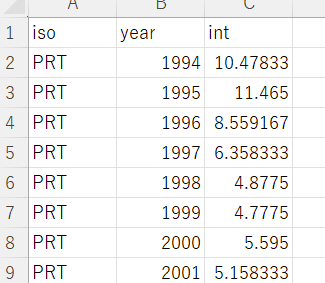and long term unpenployment data.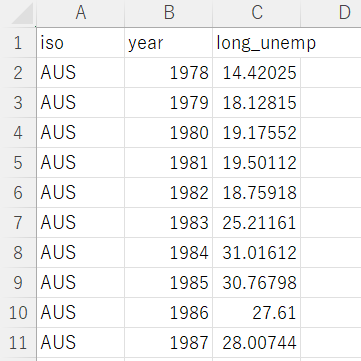longterm unenployment is "number of unenployee more than 12 months / number of all unenpolyee".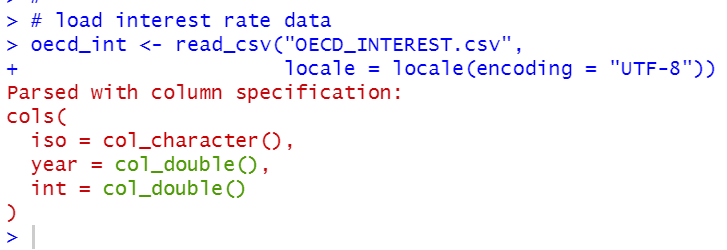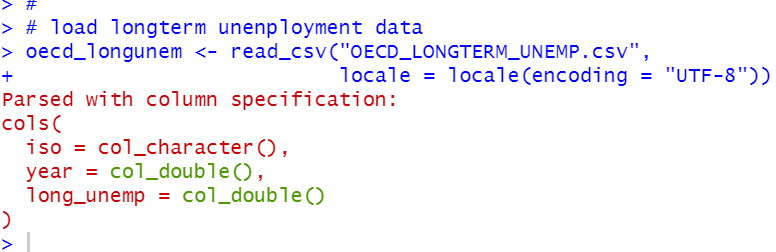Next, I used inner_join() function to merge df dataframe and the two data frames.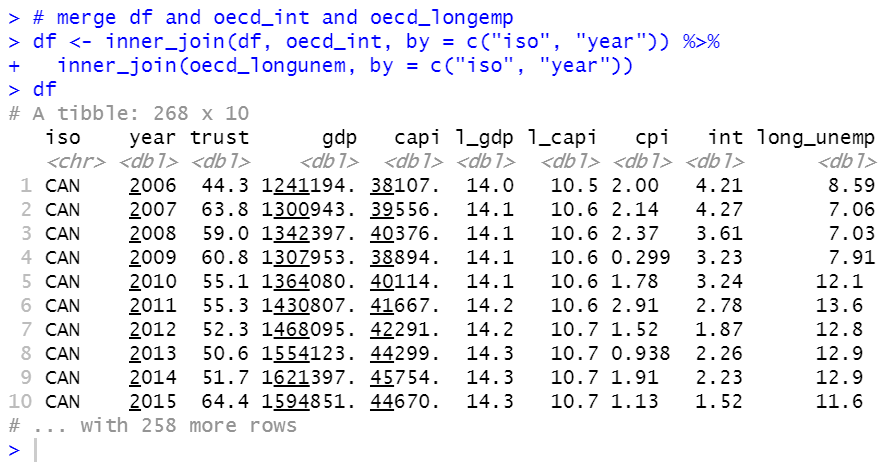Then, I will use iso = JPN only because I am a Japanese and I have special interest to Japan's Trust in gorvenment.

I make df_jpn with filter() function.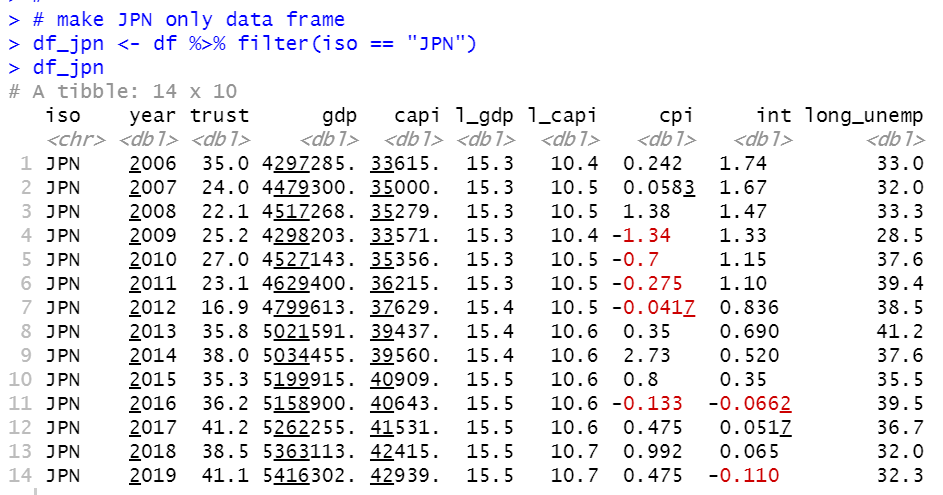Let's see scatter plot for trust, l_capi, cpi, int and long_unem.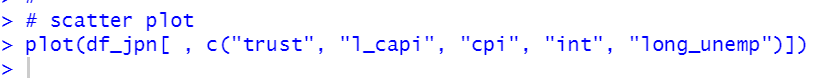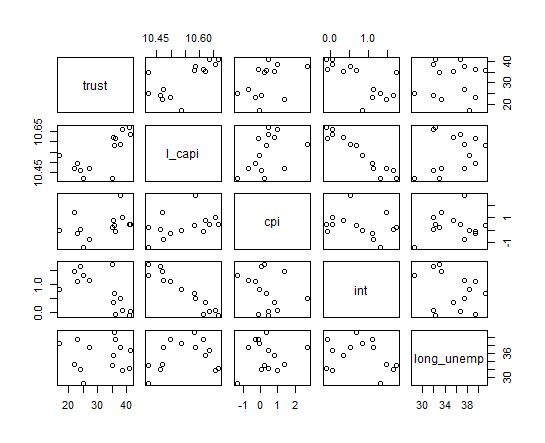Let's see correlations with cor() function.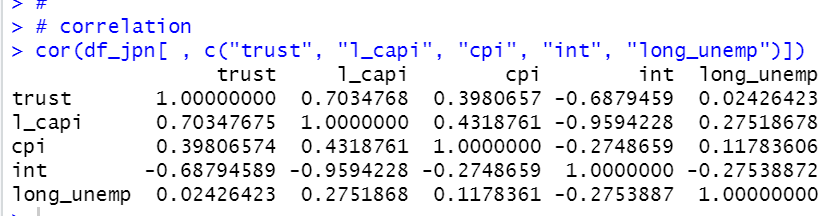I see l_capi and int has very strong negative correlation. So I will not use int for regression analysis.

Let's make a liner regression object with lm() function.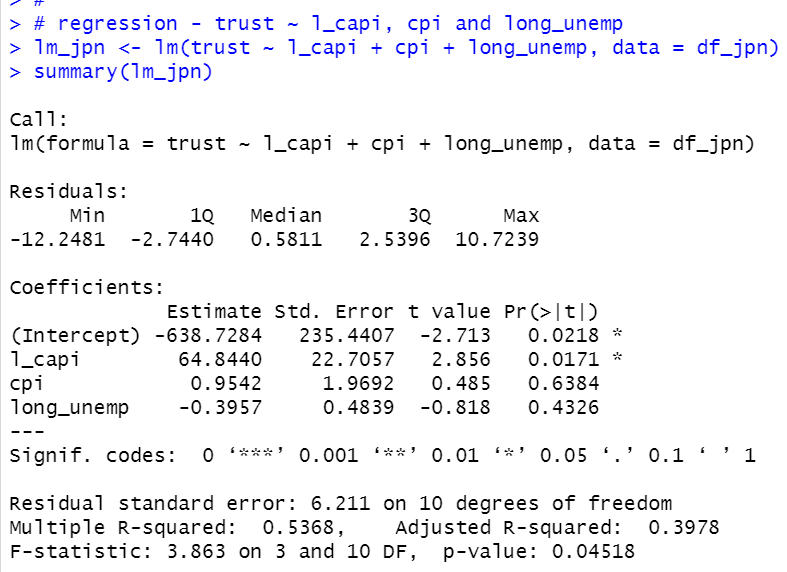coefficients of l_capi is 64.8440 and it is statistically significant.

Let's see residual plot.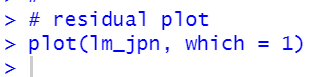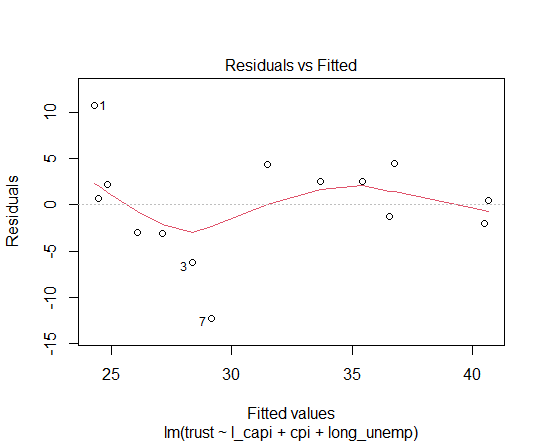Let's check if there is heteroskedasticity.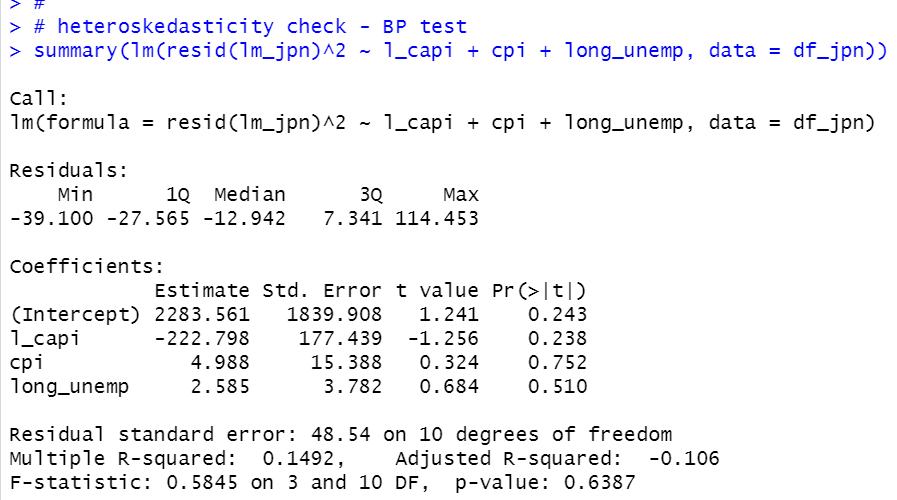There is no p-value less than 0.05. So, lm_jpn model has not heteroskedasticity.

That's it. Thank you!

Next post is